# GravitationPage 3

#### WATCH ALL SLIDES

Slide 21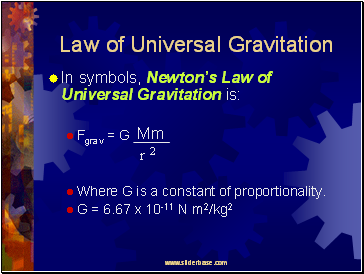## Law of Universal Gravitation

In symbols, Newton’s Law of Universal Gravitation is:

Fgrav = G

Where G is a constant of proportionality.

G = 6.67 x 10-11 N m2/kg2

Mm

r 2

Slide 22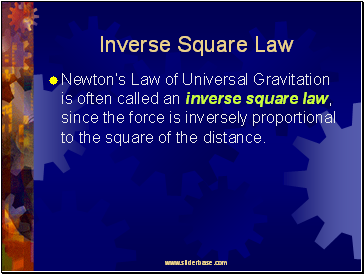## Inverse Square Law

Newton’s Law of Universal Gravitation is often called an inverse square law, since the force is inversely proportional to the square of the distance.

Slide 23An Inverse-Square Force

Slide 24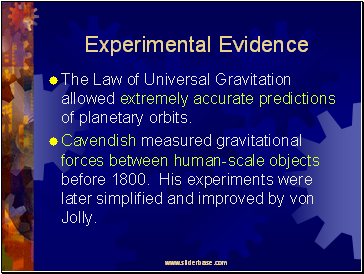Experimental Evidence

The Law of Universal Gravitation allowed extremely accurate predictions of planetary orbits.

Cavendish measured gravitational forces between human-scale objects before 1800. His experiments were later simplified and improved by von Jolly.

Slide 25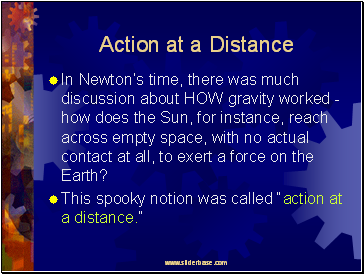## Action at a Distance

In Newton’s time, there was much discussion about HOW gravity worked - how does the Sun, for instance, reach across empty space, with no actual contact at all, to exert a force on the Earth?

This spooky notion was called “action at a distance.”

Slide 26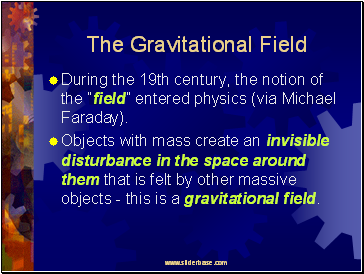## The Gravitational Field

During the 19th century, the notion of the “field” entered physics (via Michael Faraday).

Objects with mass create an invisible disturbance in the space around them that is felt by other massive objects - this is a gravitational field.

Slide 27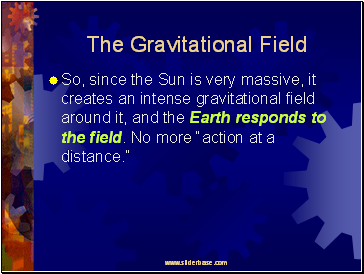The Gravitational Field

So, since the Sun is very massive, it creates an intense gravitational field around it, and the Earth responds to the field. No more “action at a distance.”

Slide 28## Gravitational Field Strength

To measure the strength of the gravitational field at any point, measure the gravitational force, F, exerted on any “test mass”, m.

Gravitational Field Strength, g = F/m

Slide 29Gravitational Field Strength

Near the surface of the Earth, g = F/m = 9.8 N/kg = 9.8 m/s2.

In general, g = GM/r2, where M is the mass of the object creating the field, r is the distance from the object’s center, and G = 6.67 x10-11 Nm2/kg2.

Slide 30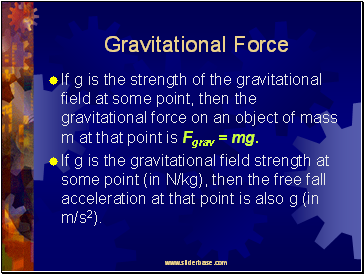## Gravitational Force

If g is the strength of the gravitational field at some point, then the gravitational force on an object of mass m at that point is Fgrav = mg.

Go to page:
1  2  3  4  5